Author: Oscar Cronquist Article last updated on May 04, 2022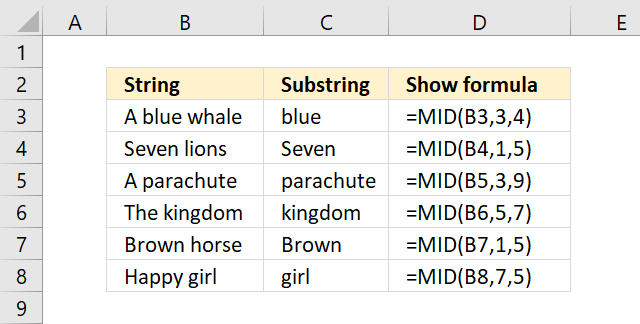The MID function returns a substring from a string based on the starting position and the number of characters you want to extract.

## 1. Excel Function Syntax

MID(text, start_num, num_chars)

## 2. Arguments

 text Text string or a cell reference to a text string. start_num The starting point. num_chars The number of characters you want to extract.

## 3. MID function example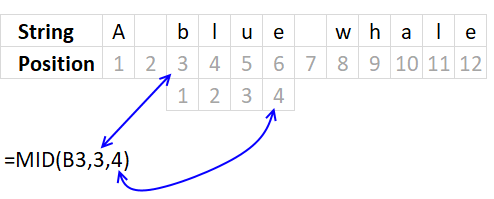The first argument is the text string, the second argument is the start position. In this case character number 3, note spaces are counted.

The third argument is how many characters you want to extract counted from the start position.

## 4. MID function from right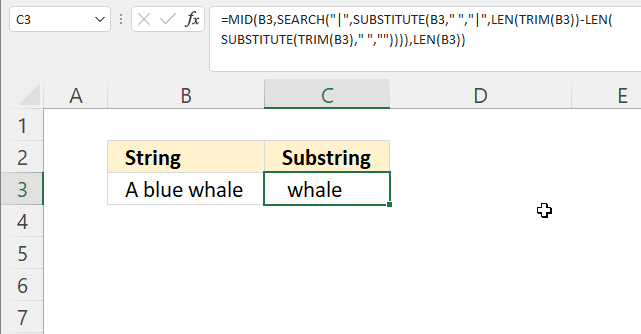The formula shown in cell C3 in the image above extracts the last word from the right based on the last space character.

Formula in cell C3:

=MID(TRIM(B3), SEARCH("|", SUBSTITUTE(B3, " ", "|", LEN(TRIM(B3))-LEN(SUBSTITUTE(TRIM(B3), " ", "")))), LEN(B3))

### 4.1 Explaining formula

#### Step 1 - Remove space charcaters

The TRIM function removes leading and trailing space characters.

TRIM(B3)

becomes

TRIM("A blue whale")

and returns "A blue whale".

#### Step 2 - Replace space character

The SUBSTITUTE function allows you to replace a given string of characters.

SUBSTITUTE(text, old_text, new_text, [instance_num])

SUBSTITUTE(TRIM(B3), " ", "")

becomes

SUBSTITUTE("A blue whale", " ", "")

and returns "Abluewhale".

#### Step 3 - Count characters

The LEN function returns a number representing the number of characters in a given string.

LEN(SUBSTITUTE(TRIM(B3), " ", ""))

becomes

LEN("Abluewhale")

and returns 10.

#### Step 4 - Subtract total character length with string without space characters.

The minus character lets you subtract numbers in an Excel formula.

LEN(TRIM(B3))-LEN(SUBSTITUTE(TRIM(B3), " ", ""))

becomes

12-10 equals 2. There are two space characters in the string.

#### Step 5 - Replace last space with | character

SUBSTITUTE(B3, " ", "|", LEN(TRIM(B3))-LEN(SUBSTITUTE(TRIM(B3), " ", "")))

becomes

SUBSTITUTE(B3, " ", "|", 2)

becomes

SUBSTITUTE("A blue whale", " ", "|", 2)

and returns "A blue|whale".

#### Step 6 - Find | character in string

The SEARCH function returns a number representing the position of character at which a specific text string is found reading left to right.

SEARCH(find_text,within_text, [start_num])

SEARCH("|", SUBSTITUTE(B3, " ", "|", LEN(TRIM(B3))-LEN(SUBSTITUTE(TRIM(B3), " ", ""))))

becomes

SEARCH("|", "A blue|whale")

and returns 7. Character | is in the seventh positiion in the string.

#### Step 7 - Extract last word

MID(TRIM(B3), SEARCH("|", SUBSTITUTE(B3, " ", "|", LEN(TRIM(B3))-LEN(SUBSTITUTE(TRIM(B3), " ", "")))), LEN(B3))

becomes

MID(TRIM(B3), 7, LEN(B3))

becomes

MID(TRIM(B3), 7, 12)

becomes

MID("A blue whale", 7, 12)

and returns "whale".

## 5. MID function with find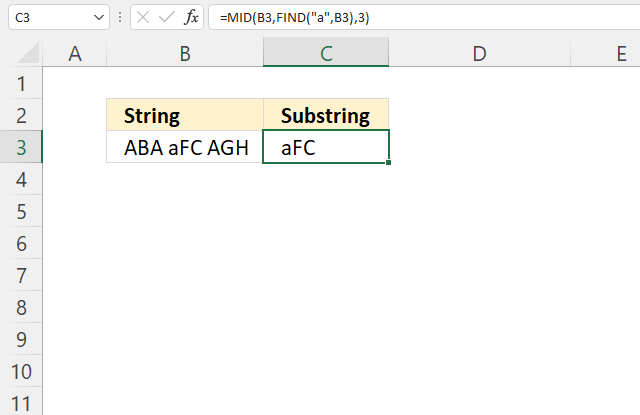The formula in cell C3 uses the FIND function to search for a string also considering upper and lower letters as well.

Formula in cell C3:

=MID(B3, FIND("a", B3), 3)

### 5.1 Explaining formula

#### Step 1 - Find position of a given string

The FIND function returns the position of a specific string in another string, reading left to right. Note, the FIND function is case-sensitive.

FIND(find_text,within_text, [start_num])

FIND("a", B3)

becomes

FIND("a", "ABA aFC AGH")

and returns 5.

#### Step 2 - Extract three characters based on position of a given string

MID(B3, FIND("a", B3), 3)

becomes

MID(B3, 5, 3)

becomes

MID("ABA aFC AGH", 5, 3)

and returns "aFC".

## 6. MID function with search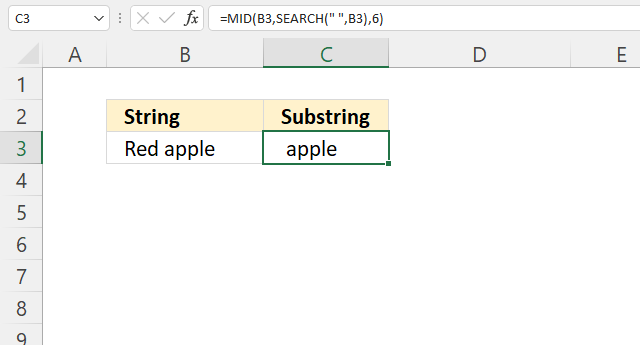The formula in cell C3 extracts a substring based on a character position. The position is calculated using the SEARCH function.

Formula in cell C3:

=MID(B3, SEARCH(" ", B3), 6)

### 6.1 Explaining formula

#### Step 1 - Find space charcater position in string

The SEARCH function returns a number representing the position of character at which a specific text string is found reading left to right.

SEARCH(find_text,within_text, [start_num])

SEARCH(" ", B3)

becomes

SEARCH(" ", "Red apple")

and returns 4.

#### Step 2 - Extract substring based on position

MID(B3, SEARCH(" ", B3), 6)

becomes

MID(B3, 4, 6)

becomes

MID("Red apple", 4, 6)

and returns "apple".

## 7. MID function between two characters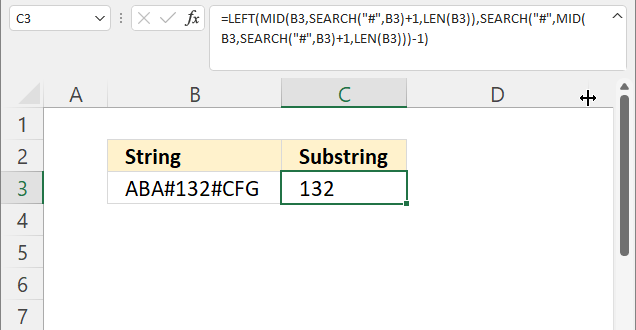The formula in cell C3 extracts the characters between two given characters, in this example, the hastag character.

Formula in cell C3:

=LEFT(MID(B3, SEARCH("#", B3)+1, LEN(B3)), SEARCH("#", MID(B3, SEARCH("#", B3)+1, LEN(B3)))-1)

### 7.1 Explaining formula

#### Step 1 - Find character in string

The SEARCH function returns a number representing the position of the character at which a specific text string is found reading left to right.

SEARCH(find_text,within_text, [start_num])

SEARCH("#", B3)+1

becomes

4+1 equals 5.

#### Step 2 - Count characters in cell

The LEN function returns a number representing the number of characters in a given string.

LEN(value)

LEN(B3)

becomes

LEN("ABA#132#CFG")

and returns 11.

#### Step 3 - Extract string

MID(B3, SEARCH("#", B3)+1, LEN(B3))

becomes

MID(B3, 5, LEN(B3))

becomes

MID(B3, 5, 11)

becomes

MID("ABA#132#CFG", 5, 11)

and returns "132#CFG".

#### Step 4 - Find second instance of character

SEARCH("#", MID(B3, SEARCH("#", B3)+1, LEN(B3)))-1

becomes

SEARCH("#", "132#CFG")-1

becomes

4-1 equals 3.

#### Step 5 - Extract string

LEFT(MID(B3, SEARCH("#", B3)+1, LEN(B3)), SEARCH("#", MID(B3, SEARCH("#", B3)+1, LEN(B3)))-1)

becomes

LEFT("132#CFG", SEARCH("#", MID(B3, SEARCH("#", B3)+1, LEN(B3)))-1)

becomes

LEFT("132#CFG", 3)

and returns "132".

## 8. MID function - split characters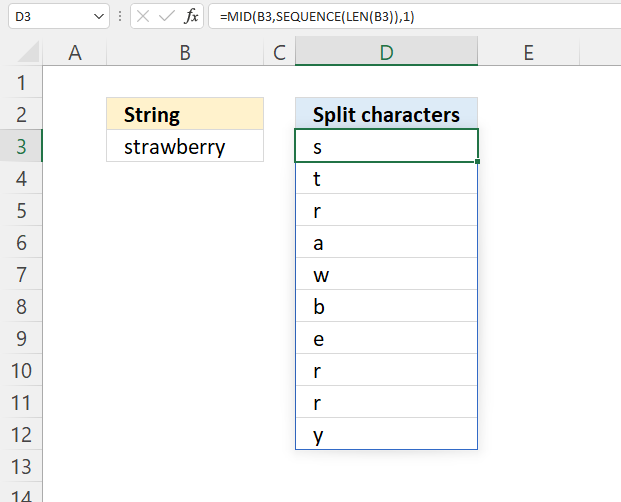This example demonstrates a formula that splits each character into a vertical array, there is also a formula below that splits characters horizontally.

Formula in cell D3:

=MID(B3, SEQUENCE(LEN(B3)), 1)

### 8.1 Explaining formula

#### Step 1 - Count characters in cell B3

The LEN function returns a number representing the number of characters in a given string.

LEN(value)

LEN(B3)

becomes

LEN("strawberry")

and returns 10. There are ten characters in cell B3.

#### Step 2 - Create a list of numbers

The SEQUENCE function creates a list of sequential numbers.

SEQUENCE(rows, [columns], [start], [step])

SEQUENCE(LEN(B3))

becomes

SEQUENCE(10)

and returns {1; 2; 3; 4; 5; 6; 7; 8; 9; 10}.

#### Step 3 - Split characters in value

MID(B3, SEQUENCE(LEN(B3)), 1)

becomes

MID("strawberry", {1; 2; 3; 4; 5; 6; 7; 8; 9; 10}, 1)

and returns {"s"; "t"; "r"; "a"; "w"; "b"; "e"; "r"; "r"; "y"}.

Use this Excel 365 dynamic array formula to split characters horizontally:

=MID(B3, SEQUENCE(,LEN(B3)), 1)

Array formula for earlier Excel versions:

=MID(B3, ROW(\$A\$1:INDEX(\$A:\$A, LEN(B3))), 1)

## 9. MID function - reverse characters in a value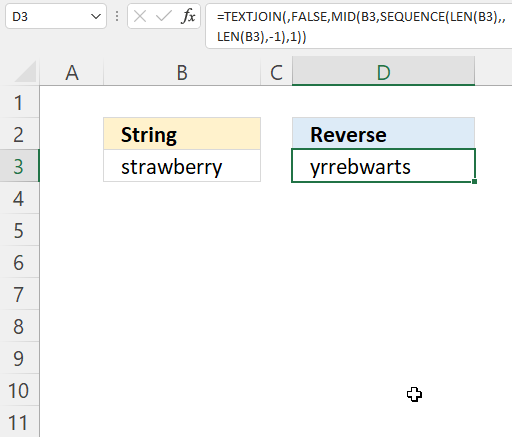Excel 365 dynamic array formula in cell D3:

=TEXTJOIN(, FALSE, MID(B3, SEQUENCE(LEN(B3), , LEN(B3), -1), 1))

### Explaining formula

#### Step 1 - Count characters in cell B3

The LEN function returns a number representing the number of characters in a given string.

LEN(value)

LEN(B3)

becomes

LEN("strawberry")

and returns 10.

#### Step 2 - Count characters in cell B3

The SEQUENCE function creates a list of sequential numbers.

SEQUENCE(rows, [columns], [start], [step])

SEQUENCE(LEN(B3), , LEN(B3), -1)

becomes

SEQUENCE(10, , 10, -1)

and returns {10; 9; 8; 7; 6; 5; 4; 3; 2; 1}.

#### Step 3 - Split characters in value

MID(B3, SEQUENCE(LEN(B3), , LEN(B3), -1), 1)

becomes

MID(B3, {10; 9; 8; 7; 6; 5; 4; 3; 2; 1}, 1)

becomes

MID("strawberry", {10; 9; 8; 7; 6; 5; 4; 3; 2; 1}, 1)

and returns {"y"; "r"; "r"; "e"; "b"; "w"; "a"; "r"; "t"; "s"}.

#### Step 4 - Join characters

The TEXTJOIN function combines text strings from multiple cell ranges.

TEXTJOIN(delimiterignore_emptytext1[text2], ...)

TEXTJOIN(, FALSE, MID(B3, SEQUENCE(LEN(B3), , LEN(B3), -1), 1))

becomes

TEXTJOIN(, FALSE, {"y"; "r"; "r"; "e"; "b"; "w"; "a"; "r"; "t"; "s"})

and returns "yrrebwarts".